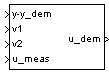# 2D Observer Form [A(v),B(v),C(v),F(v),H(v)]

Implement gain-scheduled state-space controller in observer form depending on two scheduling parameters

• Library:
• Aerospace Blockset / GNC / Control

•## Description

The 2D Observer Form [A(v),B(v),C(v),F(v),H(v)] block implements a gain-scheduled state-space controller as defined in Algorithms.

The output from this block is the actuator demand, which you can input to an actuator block. Use this block to implement a controller designed using H-infinity loop-shaping, one of the design methods supported by Robust Control Toolbox.

## Limitations

If the scheduling parameter inputs to the block go out of range, they are clipped. The state-space matrices are not interpolated out of range.

## Ports

### Input

expand all

Set-point error, specified as a vector.

Data Types: `double`

First scheduling variable, specified as a vector, that conforms to the dimensions of the state-space matrices.

Data Types: `double`

Second scheduling variable, specified as a vector, that conforms to the dimensions of the state-space matrices.

Data Types: `double`

Measured actuator position, specified as a vector.

Data Types: `double`

### Output

expand all

Actuator demands, specified as a vector.

Data Types: `double`

## Parameters

expand all

A-matrix of the state-space implementation. In the case of 2-D scheduling, the A-matrix should have four dimensions, the last two corresponding to scheduling variables v1 and v2. For example, if the A-matrix corresponding to the first entry of v1 and first entry of v2 is the identity matrix, then ```A(:,:,1,1) = [1 0;0 1];```.

#### Programmatic Use

 Block Parameter: `A` Type: character vector Values: vector Default: `'A'`

B-matrix of the state-space implementation. In the case of 2-D scheduling, the B-matrix should have four dimensions, the last two corresponding to scheduling variables v1 and v2. For example, if the B-matrix corresponding to the first entry of v1 and first entry of v2 is the identity matrix, then ```B(:,:,1,1) = [1 0;0 1];```.

#### Programmatic Use

 Block Parameter: `B` Type: character vector Values: vector Default: `'B'`

C-matrix of the state-space implementation. In the case of 2-D scheduling, the C-matrix should have four dimensions, the last two corresponding to scheduling variables v1 and v2. For example, if the C-matrix corresponding to the first entry of v1 and first entry of v2 is the identity matrix, then ```C(:,:,1,1) = [1 0;0 1];```.

#### Programmatic Use

 Block Parameter: `C` Type: character vector Values: vector Default: `'C'`

State-feedback matrix. In the case of 2-D scheduling, the F-matrix should have four dimensions, the last two corresponding to scheduling variables v1 and v2. For example, if the F-matrix corresponding to the first entry of v1 and first entry of v2 is the identity matrix, then `F(:,:,1,1) = [1 0;0 1];`.

#### Programmatic Use

 Block Parameter: `F` Type: character vector Values: vector Default: `'F'`

Observer (output injection) matrix. In the case of 2-D scheduling, the H-matrix should have four dimensions, the last two corresponding to scheduling variables v1 and v2. For example, if the H-matrix corresponding to the first entry of v1 and first entry of v2 is the identity matrix, then ```H(:,:,1,1) = [1 0;0 1];```.

#### Programmatic Use

 Block Parameter: `H` Type: character vector Values: vector Default: `'H'`

Vector of the breakpoints for the first scheduling variable. The length of v1 should be same as the size of the third dimension of A, B, C, F, and H.

#### Programmatic Use

 Block Parameter: `AoA_vec` Type: character vector Values: vector Default: `'v1_vec'`

Vector of the breakpoints for the second scheduling variable. The length of v2 should be same as the size of the fourth dimension of A, B, C, F, and H.

#### Programmatic Use

 Block Parameter: `Mach_vec` Type: character vector Values: vector Default: `'v2_vec'`

Vector of initial states for the controller,that is, initial values for the state vector, x. It should have length equal to the size of the first dimension of A.

#### Programmatic Use

 Block Parameter: `x_initial` Type: character vector Values: vector Default: `'0'`

## Algorithms

The block implements a gain-scheduled state-space controller defined in the following observer form:

`$\begin{array}{l}\stackrel{˙}{x}=\left(A\left(v\right)+H\left(v\right)C\left(v\right)\right)x+B\left(v\right){u}_{meas}+H\left(v\right)\left(y-{y}_{dem}\right)\\ {u}_{dem}=F\left(v\right)x\end{array}$`

 Hyde, R. A.. "H-infinity Aerospace Control Design — A VSTOL Flight Application." Advances in Industrial Control Series, Springer Verlag, 1995.

## SupportGet trial now